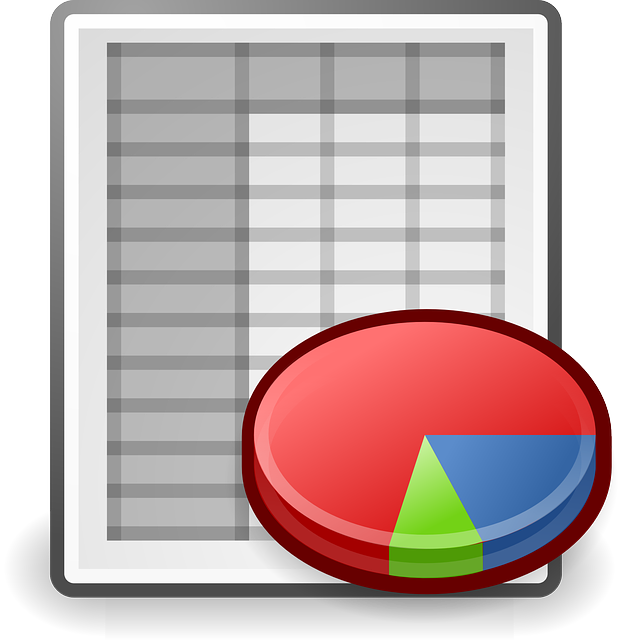# Measurement units. Practical conversion rules.

July 17, 2016 SorinMStamate

### Measurement units.

The measurement units used in marine are divided into two systems.

Imperial system (miles, cables, etc.).

For example: The mile is equal with length of arc of 1’ of the Earth’s meridian, considered as a sphere and the volume equal with the volume of terrestrial ellipsoid.

International System (kilometre, meter, etc.).

### Practical conversion rules.

In different calculations for navigation, it is necessary to convert the values of units in other units.

Conversion is easy if you know the basic relationship between the two units.

For calculation, they made special tables and settled practical conversion rules.Measurement units. Practical conversion rules _ v1.0